# 4. PTM1, C2 – Pair stats

## 4.1 – Correlation and its types

I have to mention this at this point. The pair trading technique we are discussing now is discussed in a book called, ‘Trading Pairs’, by Mark Whistler. I like this book for the fact that it got me hooked to Pair trading and over time as my interest grew, I explored the strategy beyond Mark Whistler’s techniques. Needless to say, I will discuss those techniques later in this module.  At this point, my intention is to take you through the exact learning path I underwent learning pair trading.

Towards the end of the previous chapter, we introduced the concept of correlation and the way one can analyze the correlation values. We will take that discussion forward now and understand how to calculate the correlation between two stocks, on excel. As you may have guessed by now, the calculation of Correlation between two stocks is the key in pair trading.

For the sake of this example, I’ve considered Axis Bank and ICICI Bank. Both are Private sector banks and have similar business backgrounds, hence intuition says that the two stocks should be highly correlated.

At this point, I have downloaded the closing price of Axis Bank and ICICI Bank from 4th Dec 2015 to 4th Dec 2017, roughly 2 years of trading data or about 496 data points.

Before we proceed, a quick note on data –

1. Make sure you are dealing with the same number of data points. For example, if you have 400 data point for Stock A, then you need to ensure you have the same number of data points for Stock B, corresponding to same dates.
2. Make sure the data is cleaned for corporate actions such as bonus/splits etc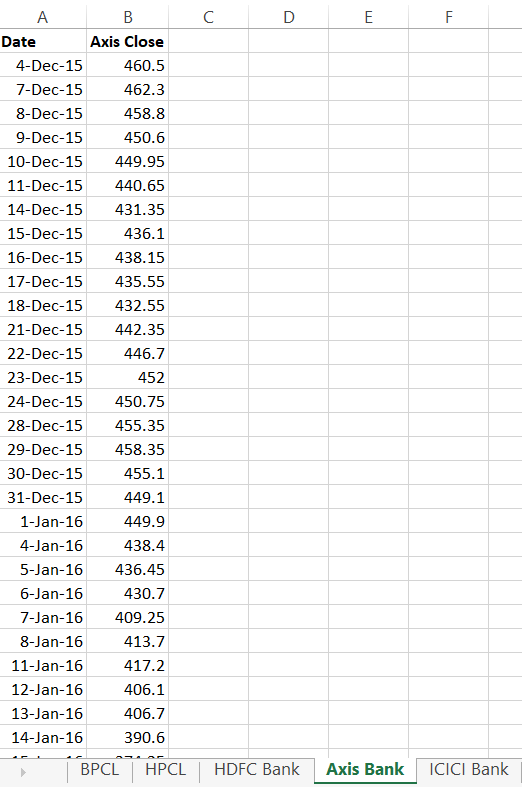As you can see from the above image, besides ICICI and Axis, I have also downloaded the data for BPCL, HPCL, and HDFC Bank. You can use this data to build and test other correlations.

Anyway, at this stage, the only data we have is the date and the closing price of the stock. We will go ahead and calculate the daily returns. I guess you are familiar with the daily return calculation, we have discussed this several time in the previous module.

The daily return can be calculated as

= [today’s closing price / previous day’s closing price] – 1

I’ve calculated this for both ICICI and Axis Bank –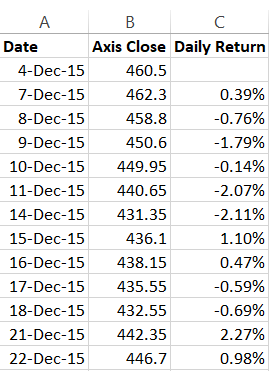Now, correlation can be calculated on the basis of two parameters –

1. The daily closing price
2. The daily return series

The daily closing price correlation requires you to calculate the correlation based on the closing prices of two stock. I’m not a big fan of calculating correlation on closing prices, but then let’s just go ahead and do this for time being.

To do this in excel, simply use the ‘=Correl()’, function on the daily closing prices. I’m running this calculation on a new sheet, which is labeled it as ‘Pair Data’.

Here is the snapshot –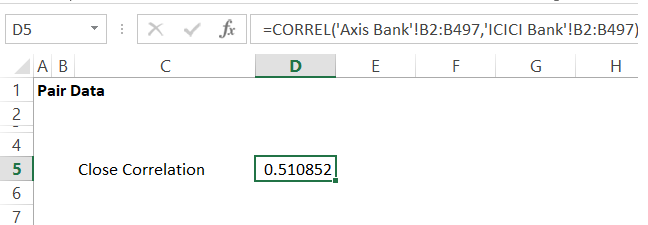The correlation between the closing prices of ICICI Bank and Axis bank is 0.51. Not particularly a great correlation, but we can live with this for now. Do recollect, our gut said the two banks could be highly correlated as they have similar business backgrounds, but the number is painting a slightly different picture 🙂

We will now run the correlation on the daily % return series for the two stock. I’ve already calculated this % return, I’ll just have to run the correl function now.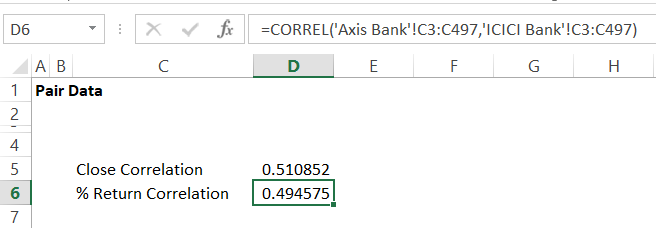Again, not a very encouraging number, but that is ok for now.

Some traders, run the correlation on the absolute per day change calculated as ‘Today’s stock price – yesterday’s stock price’.  Again, I’m not a big fan of this. But let me just go ahead and introduce the same to you –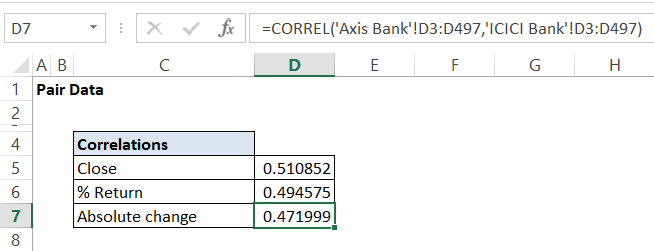In all the above calculations, I’ve run the correlation of Axis Bank versus ICICI Bank, the results obtained will be same if I had opted to calculate the correlation of ICIC Bank versus Axis. Generally speaking, the correlation between A and B is the same as Correlation between B and A.

In this method of trading pairs, the correlation number is considered sacred. Ideally speaking, the number should be above 0.75. Clearly, that is not the case with ICICI and Axis, but then as I mentioned earlier, we can live with it.

## 4.2 – Setting up the datasheet

In the previous chapter, we discussed three variables concerning the pairs namely the spread, differential, and the ratios. Let us go ahead and calculate these variables on the two stocks we are studying. We will do this on a separate sheet within the same workbook and name the sheet as the ‘Data Sheet’. Here is the snapshot –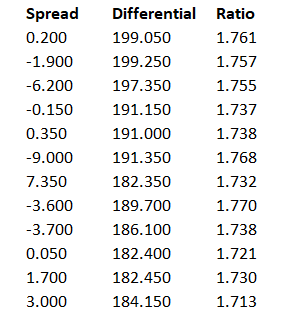The calculation of these variables is quite straightforward, I’ve explained this in the previous chapter.

Different types of Pair Trading works at different complexities levels.  We will deal with basic stats for this version of pair trading. Given this, we will now define 3 most commonly used statistic variables.

## 4.3 – Basic stats

I’ll discuss 3 basic statistical terms at this stage. These are basic terms which play a very crucial role in pair trading. I’m fairly certain that you’d have learned these in your high school math, even otherwise this is quite basic and you can pick it up anytime.

To help you understand these jargons better, I’ve come up with a set of arbitrary runs scored by batsmen across 10 cricket matches –

Match Runs scored
1 72
2 65
3 44
4 100
5 82
6 55
7 100
8 23
9 51
10 34

Mean – Also called the arithmetic average, represents the average of a set of numbers. You can calculate the average by taking the sum of all the observations by the total number of observations.

So if I were to find the average in the above example, I’d total up all the scores and divide it by 10 (10 being the total number of observations).

Mean (Average) = 626/10

=62.6

On excel, you can simply use the ‘=Average ()’ function to calculate the average of any set of numbers.

Median – The median number represents the middle number of the data series when the data series is arranged in its numerical order. If there are even set of numbers (which is the case here), then we have to take the average of the middle two numbers to calculate the mean. However, if there are an odd number of data points, then we simply take the middle data point as the median.

So let me rearrange the data points in its numerical order –

23, 34, 44, 51, 55, 65, 72, 82, 100, 100

Since there are even numbers of observation, I’ll take the middle two numbers i.e 55 and 65, their average represents the median.

Median = (55 + 65)/2

=60

The excel function to calculate median is ‘=Median()’.

The mean and median when viewed together gives a sense of the trend. More on this later.

Mode – The mode of a data series is simply that data point which occurs the most number of times in the series. Clearly, 100 is repeating twice, with no other number appearing more than once, and that makes it the mode of the data series.

The excel function to calculate Mode is ‘=Mode()’.

In the next chapter, we will use these function in excel and understand its relevance to pair trading.

Stay tuned.

### Key takeaways from this chapter

1. Care has to be taken to ensure the data is clean and adjusted for corporate actions
2. Close correlation is the correlation when calculated on the closing prices of stocks
3. The % return correlation is the correlation when calculated on the daily returns of the stock
4. Mean is the arithmetic average of the data series
5. Median is the middle observation of a data series.
6. If the data series has even number of observations, then the median is the average of the middle two observations
7. If the data series has odd number of observations, then the median is the middle observation
8. The mode of a data series is that value which repeats the highest number of times
9. The mean and median, when viewed together to each other, offers great insight into the data trend.

1.Sujeet Raj says:

Thanks for the chapter. Hopefully the next one comes sooner as this feels being left out in the middle 🙂

Does kite give back-adjusted data? I look at weekly chart of infosys and it doesn’t feel like that. Maybe it had crashes (during the dotcom bubble and in 2002-03) but still I don’t feel like it is back adjusted. But YES Bank data is back adjusted.

Or is it that the back adjusting in Kite starts from a particular year?

Will we get an option to download the OHLC data from a chart in Kite 3.0 like there is in Pi?

•Karthik Rangappa says:

I understand, but then few readers felt that the content was too long, so trying to shorten the length of the chapter. Yes, whenever corporate action happens, the charts are cleaned up. I’m afraid there is no option to download the data from Kite, yet.

•Sujeet Raj says:

Will there be download from chart option ever available from Kite as it is from Pi?

•Karthik Rangappa says:

No, not in the near future.

2.Sashidhar. L says:

Sir do you think it would be possible for you to give a list of suggested readings at the end of every module /chapters, so as to help enthusiastic varsity readers like myself? Thanks a ton.

•Karthik Rangappa says:

Sure, makes sense.

•Sashidhar. L says:

Very excited to hear that sir. That mean you’d be adding the reading list at the end of each module?

•Karthik Rangappa says:

Yes, I’ll do that at the end of the module.

•Sashidhar. L says:

Hi sir. Sorry for asking again, but did you have time to compile the suggested reading lists for each module? It would it much appreciated. Thanks in advance.

•Karthik Rangappa says:

Not really, Shashidhar. However, if you are interested in Pair Trading, I’d sugges you read ‘Trading Pairs’, by Mark Whistler first and then read the book written by Ganapathy Vidyamurthy.

•Sashidhar. L says:

Thanks for the suggestion sir. But I have already read the book by Mark whistler, and it was really good. I just need one more book suggestion. Is there any books that deal with how to trade using news and announcements? Thanks in advance.

•Karthik Rangappa says:

Have you read the book by Alexander Elder, titled – ‘Trading for a Living’?

3.neerav1234 says:

Sir,
Which is the best source to get the data that is clean and adjusted.. and how do we know that it is adjusted and clean or not ??

•Karthik Rangappa says:

I’d suggest you subscribe to an NSE authorized data vendor for this. If you see the stock price suddenly dropping by 50% on a single day, then most likely the data is not clean.

•neerav1234 says:

Sir..
Please suggest few names of such vendors which are reliable from your experience..

•Karthik Rangappa says:

Check out ‘True Data’, I’ve heard they are quite good.

4.Nikhil says:

Hello Karthik,
In the data of BPCL, on 13-jul-17 there was -32.93% change in price and on HPCL there was -33.59% change in price. Is this data valid for calculations? I have calculated the correlation between these stocks and got answers which do not match. I have got 0.82 as close price correlation and 0.19 and 0.18 as % change and abs change correlation. When I plotted the chart of spread,differential and ratio I am getting huge spikes on top and bottom of chart near the dates where there is huge price change. What do you think went wrong??

•Karthik Rangappa says:

Ah, I’m afraid the data is not cleaned up for corporate action. Apologies for this.

•Nikhil says:

What do we have to do in that case? Should we only consider the data after there was huge change in price and calculate for the same?

•Karthik Rangappa says:

You just need to ensure the data is clean. By clean, I’m referring to the data being cleaned for splits and bonuses.

5.DIVJOT SINGH says:

HELLO SIR

I have calculated correlation between two closely related stocks pfc and rec.

closing price correlation was 0.53
and i was expecting it to be above 0.7.

Their daily return correlation turned out to be 0.39 which is shocking.

Between infratel and nifty 50, closing price correlation is 0.74 and daily return is 0.24

Dont know which figure to believe??

•Karthik Rangappa says:

Yes, correlation numbers can be quite shocking 🙂